Categories

# Sum Of Series: IIT JAM 2018 Problem 13

This problem appeared in IIT JAM 2018. This problem required some minor but very basic concepts of how to find the sum of a seris.

[h5p id="14"]

# Understand the problem

Let$a_n = n+\frac{1}{n} , n \in \mathbb{N}$. Then the sum of the series$\sum_{n=1}^{\infty} (-1)^{n+1} \frac{a_{n+1}}{n!} is$ (A)$e^{-1}-1$ (B)$e^{-1}$ (C)$1-e^{-1}$ (D)$1+e^{-1}$
##### Source of the problem
IIT JAM 2018 Problem 13
Series
Easy
##### Suggested Book
Real Analysis By S.K Mapa

Do you really need a hint? Try it first!

Consider$a_n = n + \frac{1}{n} , n \in \mathbb{N}$ We have to use$e^{x} = 1 + \frac{x}{1!} + \frac{x^{2}}{2!}+.....$ Specifically$e^{1} = 1+ \frac{1}{1!} + \frac{1}{2!}+....$ And$e^{-1} = 1 - \frac{1}{1!} + \frac{1}{2!} - \frac{1}{3!} + .......$ Do you want to play with it$\sum_{n=1}^{\infty} (-1)^{n+1} \frac{a_{n+1}}{n!} = \sum_{n=1}^{\infty} (-1)^{n+1} \frac{n+1 + \frac{1}{n+1}}{n!}$ =$\sum_{n=1}^{\infty}[ (-1)^{n+1} \frac{1}{(n-1)!} + (-1)^{n+1} \frac{1}{n!} + (-1)^{n+1} \frac{1}{(n+1)!}]$   Now we will be breaking it term by term for the ease of calculation. Can you do it from here?$\sum_{n=1}^{\infty} (-1)^{n+1} \frac{a_{n+1}}{n!}$ =$[1-\frac{1}{1!} + \frac{1}{2!} - ......] + [\frac{1}{1!} - \frac{1}{2!} + \frac{1}{3!} - .....] + [\frac{1}{2!} - \frac{1}{3!} + \frac{1}{4!} -.......]$ =$e^{-1} + [1-e^{-1}] + [e^{-1} + 1 - 1]$ =$e^{-1} + 1$ So option (D) is our required answer.

# The will look more easy if we take a look into the knowledge graph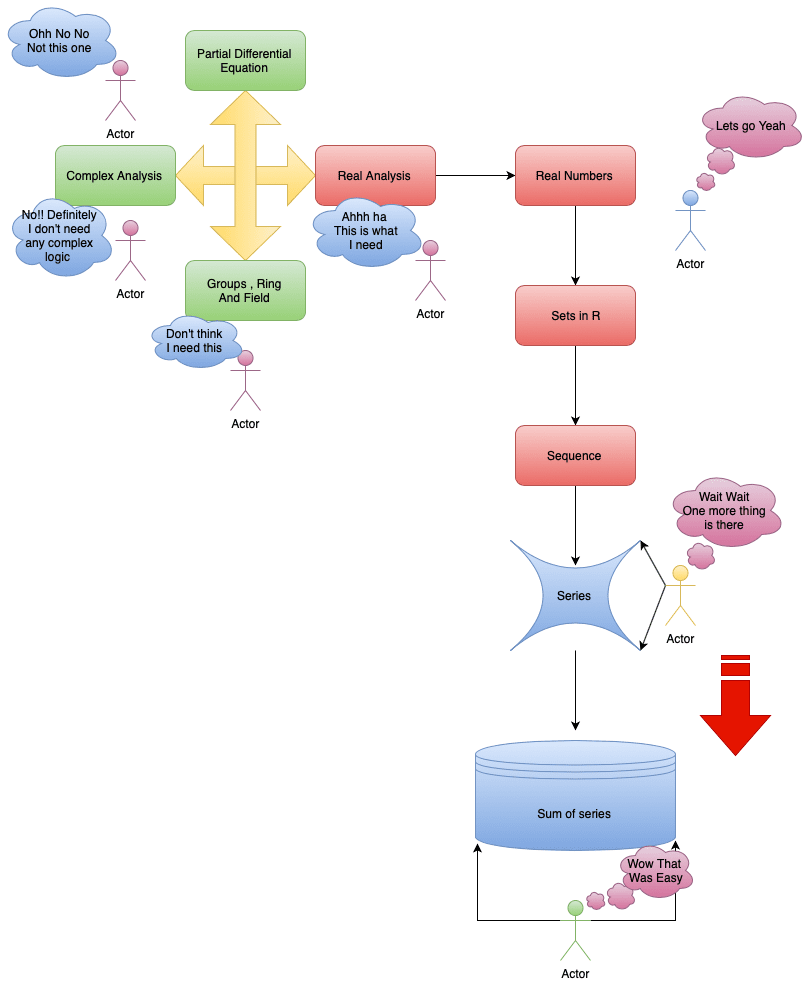# Let’s have a look into the graphs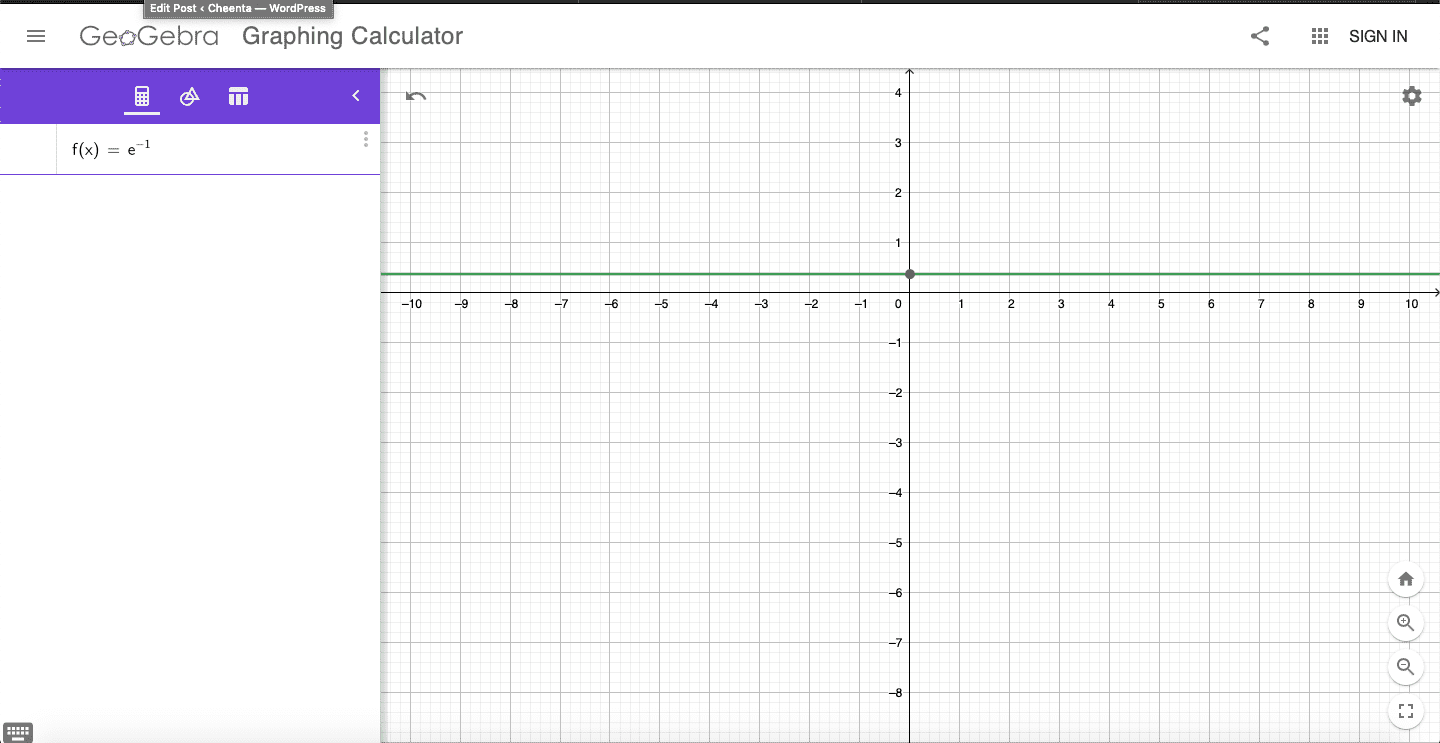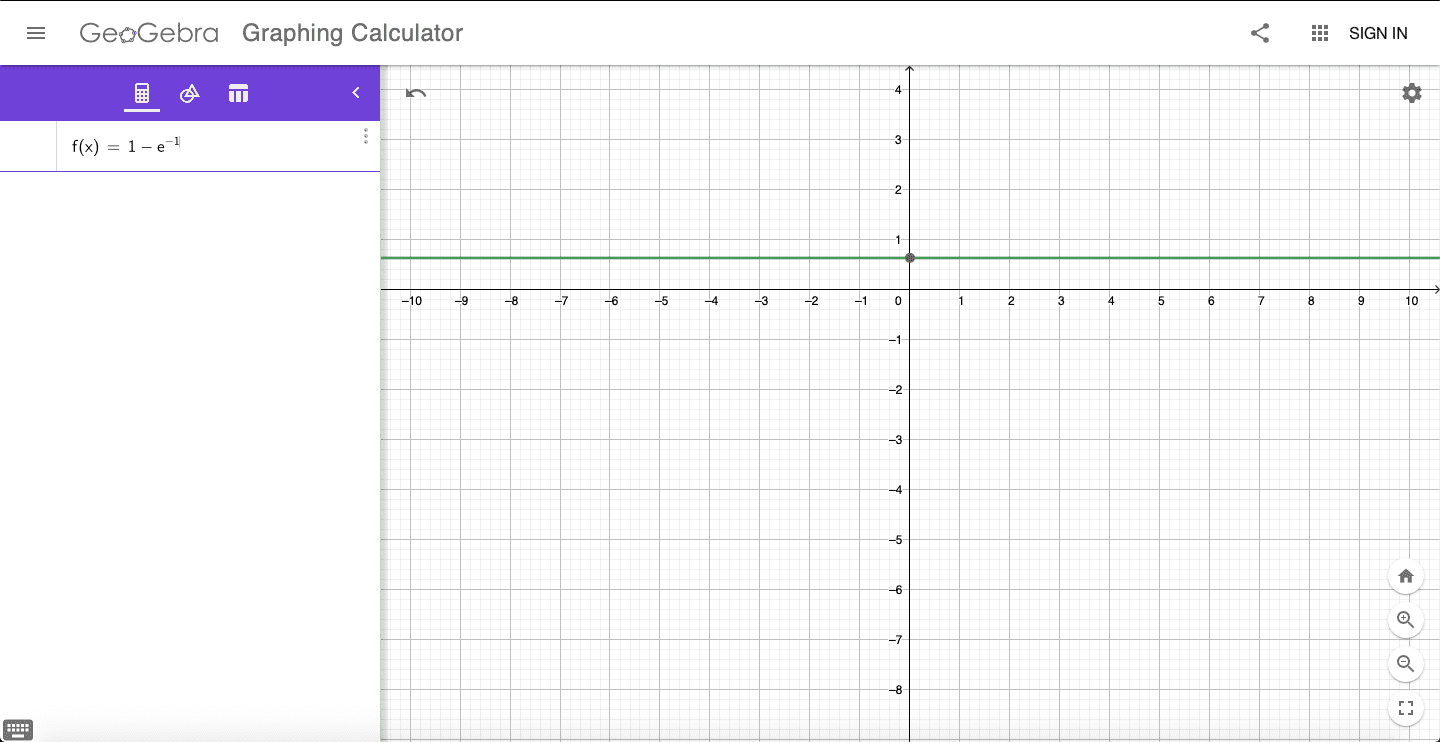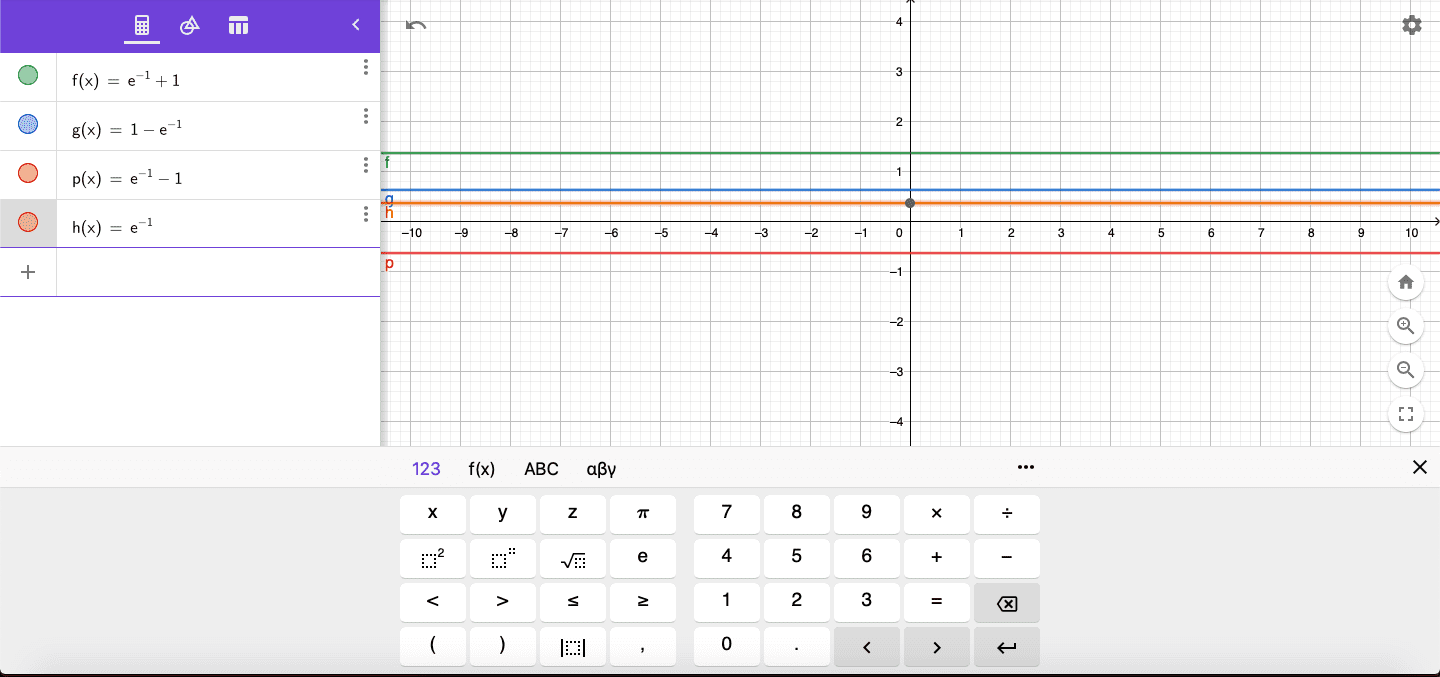# Do You Know ????# Connected Program at Cheenta

#### College Mathematics Program

The higher mathematics program caters to advanced college and university students. It is useful for I.S.I. M.Math Entrance, GRE Math Subject Test, TIFR Ph.D. Entrance, I.I.T. JAM. The program is problem driven. We work with candidates who have a deep love for mathematics. This program is also useful for adults continuing who wish to rediscover the world of mathematics.

# Similar Problems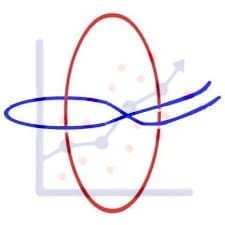## ISI MStat PSB 2006 Problem 8 | Bernoullian Beauty

This is a very simple and regular sample problem from ISI MStat PSB 2009 Problem 8. It It is based on testing the nature of the mean of Exponential distribution. Give it a Try it !## ISI MStat PSB 2009 Problem 8 | How big is the Mean?

This is a very simple and regular sample problem from ISI MStat PSB 2009 Problem 8. It It is based on testing the nature of the mean of Exponential distribution. Give it a Try it !## ISI MStat PSB 2009 Problem 4 | Polarized to Normal

This is a very beautiful sample problem from ISI MStat PSB 2009 Problem 4. It is based on the idea of Polar Transformations, but need a good deal of observation o realize that. Give it a Try it !## ISI MStat PSB 2009 Problem 6 | abNormal MLE of Normal

This is a very beautiful sample problem from ISI MStat PSB 2009 Problem 6. It is based on the idea of Restricted Maximum Likelihood Estimators, and Mean Squared Errors. Give it a Try it !## ISI MStat PSB 2009 Problem 3 | Gamma is not abNormal

This is a very simple but beautiful sample problem from ISI MStat PSB 2009 Problem 3. It is based on recognizing density function and then using CLT. Try it !## ISI MStat PSB 2009 Problem 1 | Nilpotent Matrices

This is a very simple sample problem from ISI MStat PSB 2009 Problem 1. It is based on basic properties of Nilpotent Matrices and Skew-symmetric Matrices. Try it !## ISI MStat PSB 2006 Problem 2 | Cauchy & Schwarz come to rescue

This is a very subtle sample problem from ISI MStat PSB 2006 Problem 2. After seeing this problem, one may think of using Lagrange Multipliers, but one can just find easier and beautiful way, if one is really keen to find one. Can you!

## Problem on Inequality | ISI – MSQMS – B, 2018 | Problem 2a

Try this problem from ISI MSQMS 2018 which involves the concept of Inequality. You can use the sequential hints provided to solve the problem.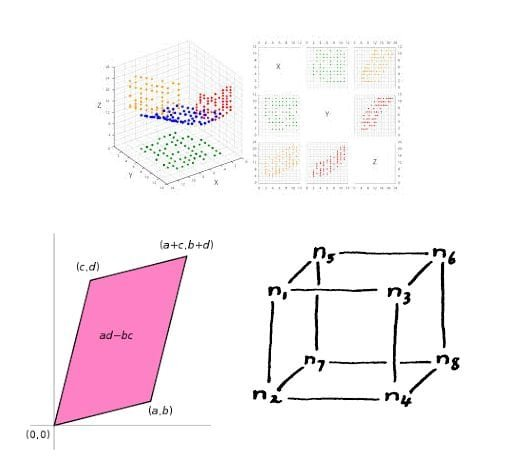## Data, Determinant and Simplex

This problem is a beautiful problem connecting linear algebra, geometry and data. Go ahead and dwelve into the glorious connection.

## Problem on Integral Inequality | ISI – MSQMS – B, 2015

Try this problem from ISI MSQMS 2015 which involves the concept of Integral Inequality and real analysis. You can use the sequential hints provided to solve the problem.

This site uses Akismet to reduce spam. Learn how your comment data is processed.